# Power Density in Biological and Astronomical Systems

Power density in various systems in the universe for a comparison of their thermodynamics efficiency

Data includes the computed metabolic rate across the ranges of species (Prokaryotes, Protists, Metazoans) and the estimated power (energy rate) density in biological and astronomical systems for a comparison of their thermodynamics efficiency.

## Examples

### Basic Examples

Retrieve the resource:

 In:=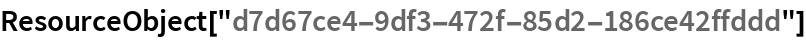Out=Retrieve the default content:

 In:=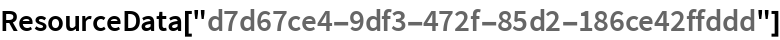Out=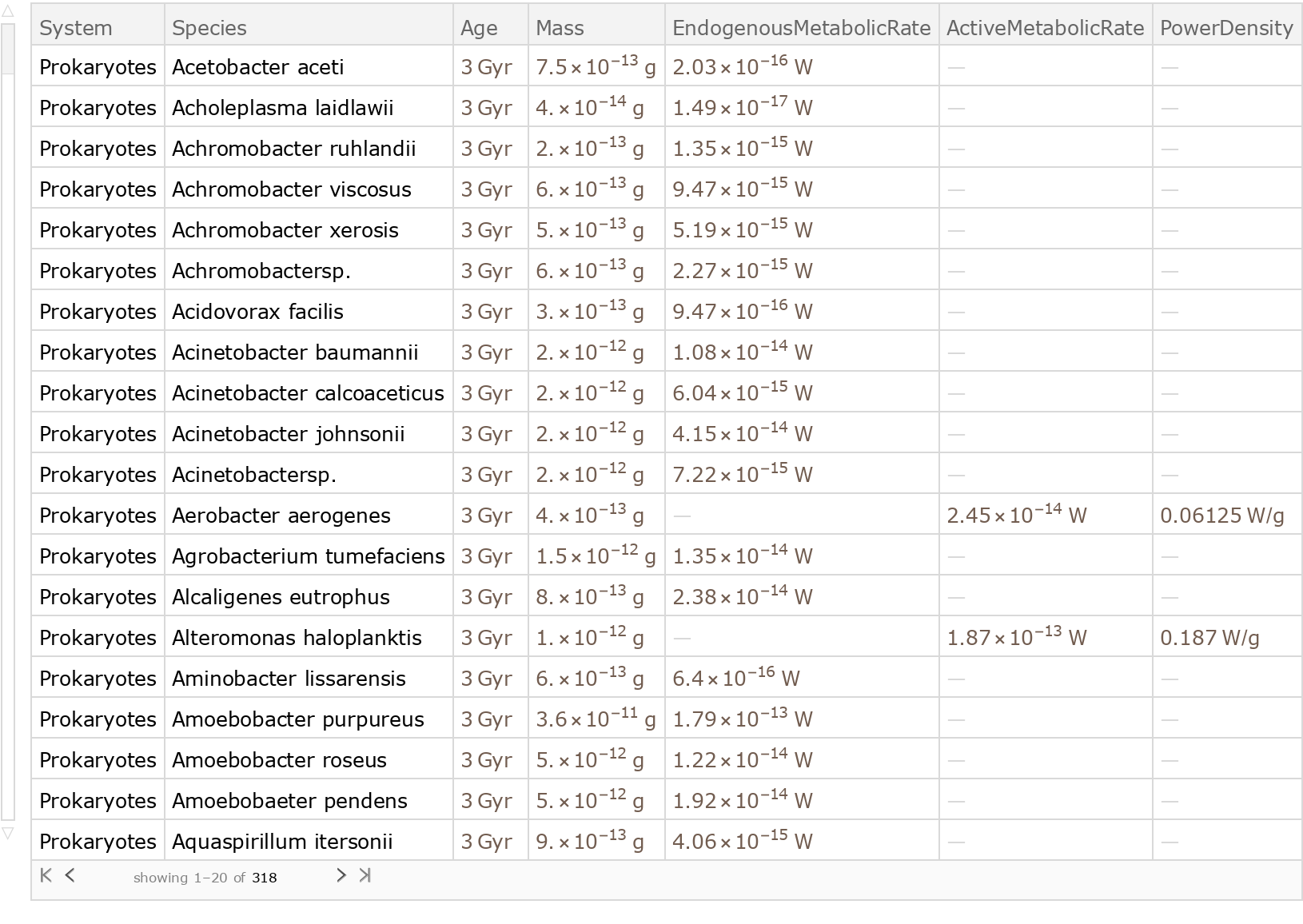Plot the power density of various systems (biological/astronomical) in the universe as a function of their age (from their origin):

 In:=Out=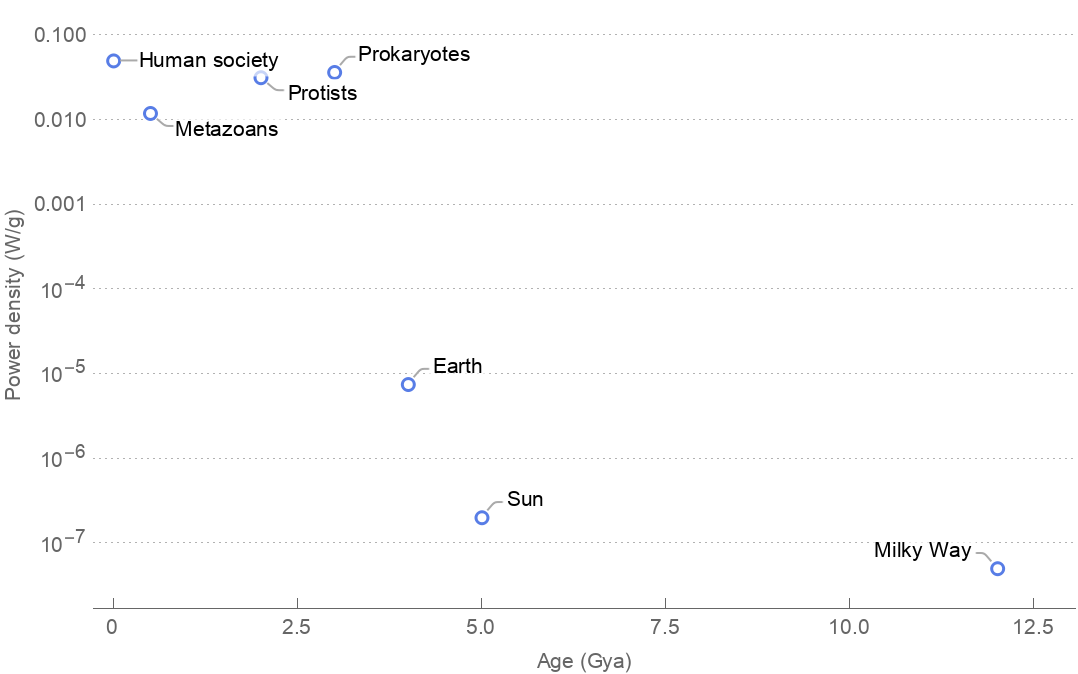Plot the relationship between mass and endogenous/active metabolic rates for Protists species:

 In:=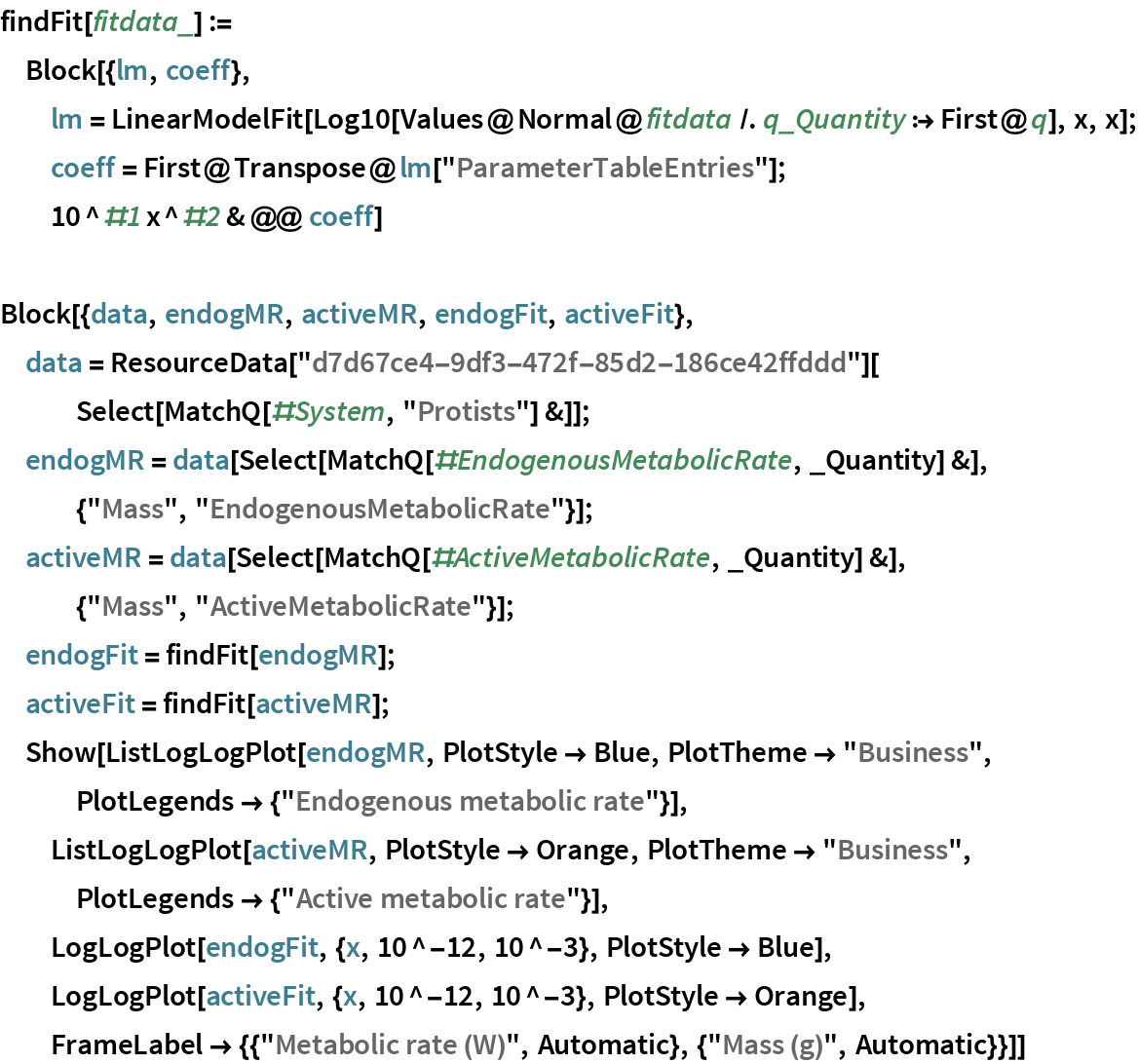Out=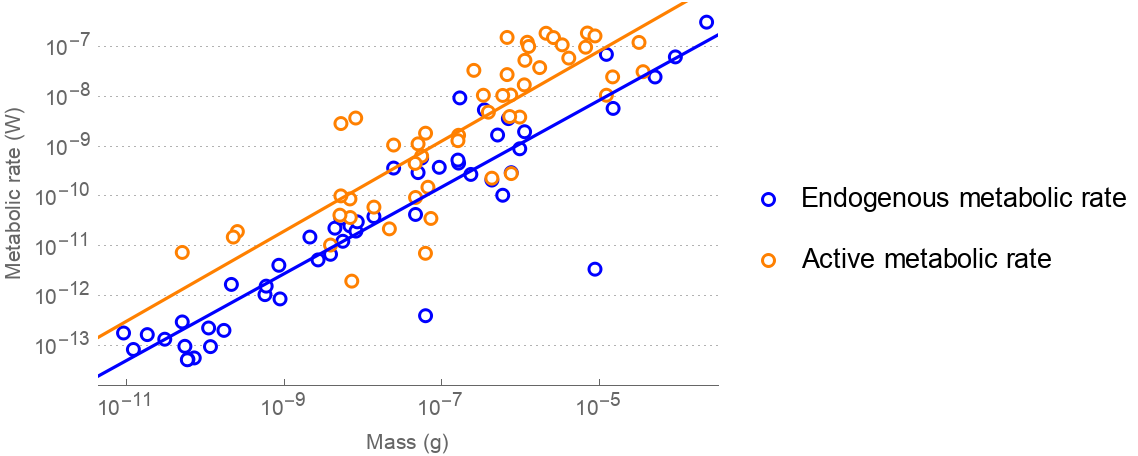Wolfram Research, "Power Density in Biological and Astronomical Systems" from the Wolfram Data Repository (2017) https://doi.org/10.24097/wolfram.08307.data